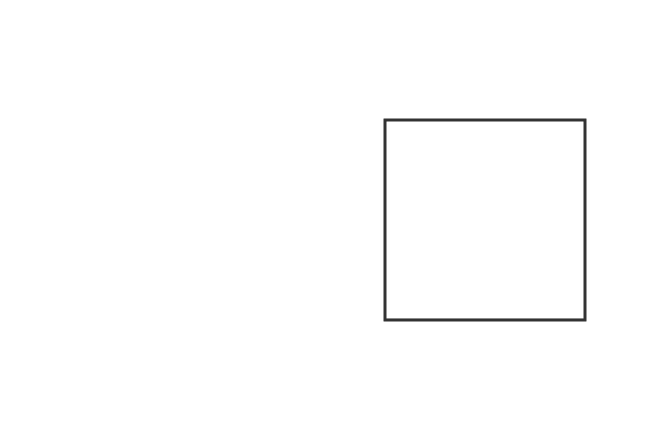# Proper fractions

A fraction whose numerator is less than its denominator is called a proper fraction.

## Introduction

The meaning of proper fraction is defined from the meanings of the words “Proper” and “Fraction”.

1. The “Proper” means right, acceptable, true, appropriate, and so on.
2. The “Fraction” means a part of the whole quantity.

According to the meanings of both words, if a part is taken from a quantity, then it is true and acceptable that the quantity of the fraction is always less than the whole quantity. Therefore, the fraction is called as the proper fraction.

### Formula

According to the theory of the proper fractions.

$Number \, of \, Selected \, parts$ $\,<\,$ $Total \, number \, of \, Parts$

$\implies$ $\dfrac{Number \, of \, Selected \, parts}{Total \, number \, of \, Parts}$ $\,<\,$ $1$

$\,\,\, \therefore \,\,\,\,\,\,$ $Proper \, fraction$ $\,=\,$ $\dfrac{Number \, of \, Selected \, parts}{Total \, number \, of \, Parts}$ $\,<\,$ $1$

The proper fraction is calculated by comparing the fraction with the whole quantity. Actually it is done by the ratio in mathematics but it is always less than one.

### Examples

Learn the concept of proper fractions from the following understandable examples.

#### Example – 1

Take a circle and divide it into three equal parts. Now, let us evaluate the proper fractions mathematically.1. If you take only one part from three parts, then the proper fraction is written as $\large \frac{1}{3}$.
2. If you consider two parts from three parts, then the proper fraction is written as $\large \frac{2}{3}$.

It is not right to take all the parts as a fraction because the sum of all parts represent the whole quantity. Therefore, the proper fractions are always less than one.

The fractions $\dfrac{1}{3}$ and $\dfrac{2}{3}$ are the proper fractions but $\dfrac{3}{3}$ is not a proper fraction and it represents a whole quantity.

#### Example – 2

Take a square and divide it into four equal parts. Now, let’s calculate the proper fractions mathematically.1. If you take only one part from four parts, then the proper fraction is written as $\large \frac{1}{4}$.
2. If you consider two parts from four parts, then the proper fraction is written as $\large \frac{2}{4}$.
3. If you take three part from four parts, then the proper fraction is expressed as $\large \frac{3}{4}$.

The rational numbers $\dfrac{1}{4}$, $\dfrac{2}{4}$ and $\dfrac{3}{4}$ represent three proper fractions because each value is less than one.

Similarly, it is not acceptable to consider all the parts as a fraction because the rational number $\dfrac{4}{4}$ represents a whole quantity.

Latest Math Topics
Jun 26, 2023
Jun 23, 2023

Latest Math Problems
Jul 01, 2023
Jun 25, 2023
###### Math Questions

The math problems with solutions to learn how to solve a problem.

Learn solutions

Practice now

###### Math Videos

The math videos tutorials with visual graphics to learn every concept.

Watch now

###### Subscribe us

Get the latest math updates from the Math Doubts by subscribing us.